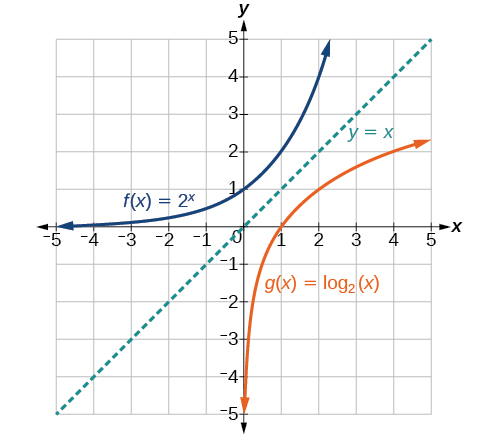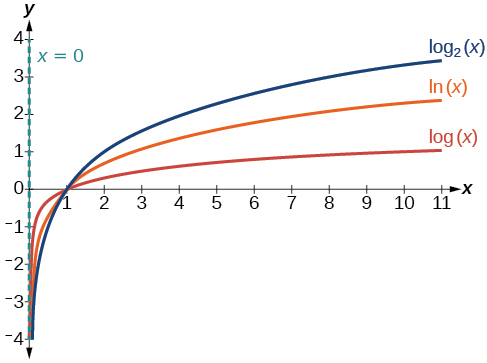# 4.4 Graphs of logarithmic functions  (Page 2/8)

 Page 2 / 8

Given a logarithmic function, identify the domain.

1. Set up an inequality showing the argument greater than zero.
2. Solve for $\text{\hspace{0.17em}}x.$
3. Write the domain in interval notation.

## Identifying the domain of a logarithmic shift

What is the domain of $\text{\hspace{0.17em}}f\left(x\right)={\mathrm{log}}_{2}\left(x+3\right)?$

The logarithmic function is defined only when the input is positive, so this function is defined when $\text{\hspace{0.17em}}x+3>0.\text{\hspace{0.17em}}$ Solving this inequality,

The domain of $\text{\hspace{0.17em}}f\left(x\right)={\mathrm{log}}_{2}\left(x+3\right)\text{\hspace{0.17em}}$ is $\text{\hspace{0.17em}}\left(-3,\infty \right).$

What is the domain of $\text{\hspace{0.17em}}f\left(x\right)={\mathrm{log}}_{5}\left(x-2\right)+1?$

$\left(2,\infty \right)$

## Identifying the domain of a logarithmic shift and reflection

What is the domain of $\text{\hspace{0.17em}}f\left(x\right)=\mathrm{log}\left(5-2x\right)?$

The logarithmic function is defined only when the input is positive, so this function is defined when $\text{\hspace{0.17em}}5–2x>0.\text{\hspace{0.17em}}$ Solving this inequality,

The domain of $\text{\hspace{0.17em}}f\left(x\right)=\mathrm{log}\left(5-2x\right)\text{\hspace{0.17em}}$ is $\text{\hspace{0.17em}}\left(–\infty ,\frac{5}{2}\right).$

What is the domain of $\text{\hspace{0.17em}}f\left(x\right)=\mathrm{log}\left(x-5\right)+2?$

$\left(5,\infty \right)$

## Graphing logarithmic functions

Now that we have a feel for the set of values for which a logarithmic function is defined, we move on to graphing logarithmic functions. The family of logarithmic functions includes the parent function $\text{\hspace{0.17em}}y={\mathrm{log}}_{b}\left(x\right)\text{\hspace{0.17em}}$ along with all its transformations: shifts, stretches, compressions, and reflections.

We begin with the parent function $\text{\hspace{0.17em}}y={\mathrm{log}}_{b}\left(x\right).\text{\hspace{0.17em}}$ Because every logarithmic function of this form is the inverse of an exponential function with the form $\text{\hspace{0.17em}}y={b}^{x},$ their graphs will be reflections of each other across the line $\text{\hspace{0.17em}}y=x.\text{\hspace{0.17em}}$ To illustrate this, we can observe the relationship between the input and output values of $\text{\hspace{0.17em}}y={2}^{x}\text{\hspace{0.17em}}$ and its equivalent $\text{\hspace{0.17em}}x={\mathrm{log}}_{2}\left(y\right)\text{\hspace{0.17em}}$ in [link] .

 $x$ $-3$ $-2$ $-1$ $0$ $1$ $2$ $3$ ${2}^{x}=y$ $\frac{1}{8}$ $\frac{1}{4}$ $\frac{1}{2}$ $1$ $2$ $4$ $8$ ${\mathrm{log}}_{2}\left(y\right)=x$ $-3$ $-2$ $-1$ $0$ $1$ $2$ $3$

Using the inputs and outputs from [link] , we can build another table to observe the relationship between points on the graphs of the inverse functions $\text{\hspace{0.17em}}f\left(x\right)={2}^{x}\text{\hspace{0.17em}}$ and $\text{\hspace{0.17em}}g\left(x\right)={\mathrm{log}}_{2}\left(x\right).\text{\hspace{0.17em}}$ See [link] .

 $f\left(x\right)={2}^{x}$ $\left(-3,\frac{1}{8}\right)$ $\left(-2,\frac{1}{4}\right)$ $\left(-1,\frac{1}{2}\right)$ $\left(0,1\right)$ $\left(1,2\right)$ $\left(2,4\right)$ $\left(3,8\right)$ $g\left(x\right)={\mathrm{log}}_{2}\left(x\right)$ $\left(\frac{1}{8},-3\right)$ $\left(\frac{1}{4},-2\right)$ $\left(\frac{1}{2},-1\right)$ $\left(1,0\right)$ $\left(2,1\right)$ $\left(4,2\right)$ $\left(8,3\right)$

As we’d expect, the x - and y -coordinates are reversed for the inverse functions. [link] shows the graph of $\text{\hspace{0.17em}}f\text{\hspace{0.17em}}$ and $\text{\hspace{0.17em}}g.$Notice that the graphs of   f ( x ) = 2 x   and   g ( x ) = log 2 ( x )   are reflections about the line   y = x .

Observe the following from the graph:

• $f\left(x\right)={2}^{x}\text{\hspace{0.17em}}$ has a y -intercept at $\text{\hspace{0.17em}}\left(0,1\right)\text{\hspace{0.17em}}$ and $\text{\hspace{0.17em}}g\left(x\right)={\mathrm{log}}_{2}\left(x\right)\text{\hspace{0.17em}}$ has an x - intercept at $\text{\hspace{0.17em}}\left(1,0\right).$
• The domain of $\text{\hspace{0.17em}}f\left(x\right)={2}^{x},$ $\left(-\infty ,\infty \right),$ is the same as the range of $\text{\hspace{0.17em}}g\left(x\right)={\mathrm{log}}_{2}\left(x\right).$
• The range of $\text{\hspace{0.17em}}f\left(x\right)={2}^{x},$ $\left(0,\infty \right),$ is the same as the domain of $\text{\hspace{0.17em}}g\left(x\right)={\mathrm{log}}_{2}\left(x\right).$

## Characteristics of the graph of the parent function, f ( x ) = log b ( x )

For any real number $\text{\hspace{0.17em}}x\text{\hspace{0.17em}}$ and constant $\text{\hspace{0.17em}}b>0,$ $b\ne 1,$ we can see the following characteristics in the graph of $\text{\hspace{0.17em}}f\left(x\right)={\mathrm{log}}_{b}\left(x\right):$

• one-to-one function
• vertical asymptote: $\text{\hspace{0.17em}}x=0$
• domain: $\text{\hspace{0.17em}}\left(0,\infty \right)$
• range: $\text{\hspace{0.17em}}\left(-\infty ,\infty \right)$
• x- intercept: $\text{\hspace{0.17em}}\left(1,0\right)\text{\hspace{0.17em}}$ and key point $\left(b,1\right)$
• y -intercept: none
• increasing if $\text{\hspace{0.17em}}b>1$
• decreasing if $\text{\hspace{0.17em}}0

[link] shows how changing the base $\text{\hspace{0.17em}}b\text{\hspace{0.17em}}$ in $\text{\hspace{0.17em}}f\left(x\right)={\mathrm{log}}_{b}\left(x\right)\text{\hspace{0.17em}}$ can affect the graphs. Observe that the graphs compress vertically as the value of the base increases. ( Note: recall that the function $\text{\hspace{0.17em}}\mathrm{ln}\left(x\right)\text{\hspace{0.17em}}$ has base $\text{\hspace{0.17em}}e\approx \text{2}.\text{718.)}$The graphs of three logarithmic functions with different bases, all greater than 1.

give me an example of a problem so that I can practice answering
x³+y³+z³=42
Robert
dont forget the cube in each variable ;)
Robert
of she solves that, well ... then she has a lot of computational force under her command ....
Walter
what is a function?
I want to learn about the law of exponent
explain this
what is functions?
A mathematical relation such that every input has only one out.
Spiro
yes..it is a relationo of orders pairs of sets one or more input that leads to a exactly one output.
Mubita
Is a rule that assigns to each element X in a set A exactly one element, called F(x), in a set B.
RichieRich
If the plane intersects the cone (either above or below) horizontally, what figure will be created?
can you not take the square root of a negative number
No because a negative times a negative is a positive. No matter what you do you can never multiply the same number by itself and end with a negative
lurverkitten
Actually you can. you get what's called an Imaginary number denoted by i which is represented on the complex plane. The reply above would be correct if we were still confined to the "real" number line.
Liam
Suppose P= {-3,1,3} Q={-3,-2-1} and R= {-2,2,3}.what is the intersection
can I get some pretty basic questions
In what way does set notation relate to function notation
Ama
is precalculus needed to take caculus
It depends on what you already know. Just test yourself with some precalculus questions. If you find them easy, you're good to go.
Spiro
the solution doesn't seem right for this problem
what is the domain of f(x)=x-4/x^2-2x-15 then
x is different from -5&3
Seid
All real x except 5 and - 3
Spiro
***youtu.be/ESxOXfh2Poc
Loree
how to prroved cos⁴x-sin⁴x= cos²x-sin²x are equal
Don't think that you can.
Elliott
By using some imaginary no.
Tanmay
how do you provided cos⁴x-sin⁴x = cos²x-sin²x are equal
What are the question marks for?
Elliott
Someone should please solve it for me Add 2over ×+3 +y-4 over 5 simplify (×+a)with square root of two -×root 2 all over a multiply 1over ×-y{(×-y)(×+y)} over ×y
For the first question, I got (3y-2)/15 Second one, I got Root 2 Third one, I got 1/(y to the fourth power) I dont if it's right cause I can barely understand the question.
Is under distribute property, inverse function, algebra and addition and multiplication function; so is a combined question
AbenaByByBy Briana HamiltonBy Anh DaoByBy Briana KnowltonBy Stephen VoronBy OpenStaxBy Carly AllenBy OpenStaxBy Sandy YamaneBy Monty Hartfield